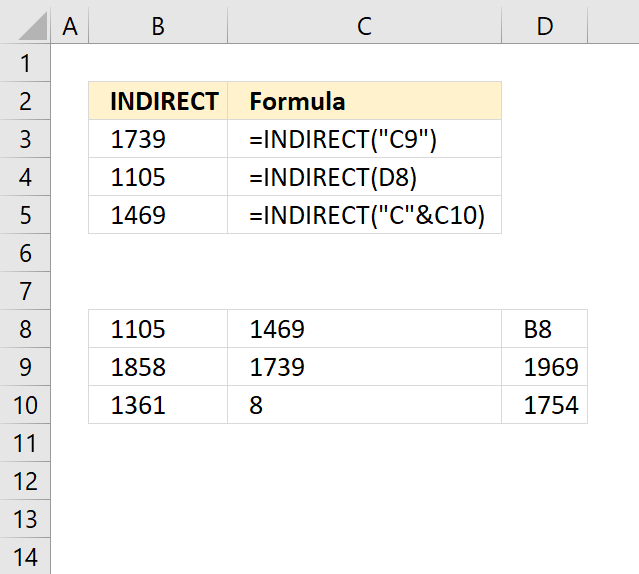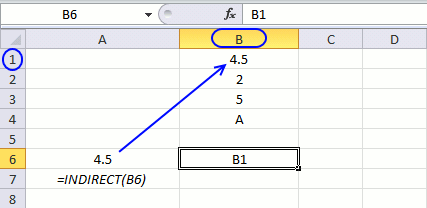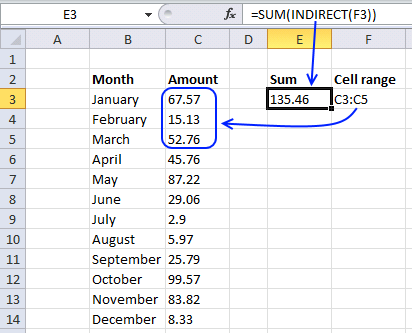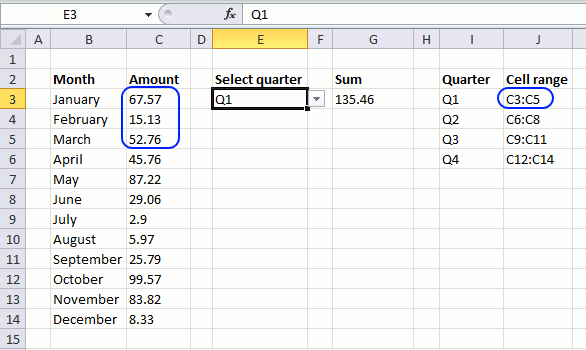Author: Oscar Cronquist Article last updated on April 17, 2018The INDIRECT function returns the cell reference based on a text string and shows the content of that cell reference.

This function is volatile, use with caution.

### Excel Function Syntax

INDIRECT(ref_text, [a1])

### Arguments

 ref_text Required. A reference to a cell, a name defined as a reference, or a reference to a cell as a text string. [a1] Optional. TRUE is the default value and is evaluated to A1- style reference. FALSE represents R1C1-style reference.

### Explaining Indirect function

This is what Microsoft tells you about the indirect function:

Cell A6 contains this formula:=INDIRECT(B6). If you enter a cell reference like B1 in cell B6, the formula in cell A6 becomes =B1 and it returns the value in cell B1. This animated picture explains it all.Can it do more than that? The answer is of course yes but first a small warning, INDIRECT is a volatile function and extended use may slower your workbook considerably.

### Example 1 - Avoid automatically changing cell references

When you insert or delete a row or column in excel the cell references in formulas changes, even if you use absolute cell references. The Indirect function helps you solve that problem. The following picture demonstrates what happens with relative, absolute and indirect cell references when you insert a row.### Example 2 - Sum a range you specifyFormula in cell E3:

=SUM(INDIRECTF3))

Cell A1 contains C3:C5. Sum function sums values in cell C3:C5. If you change the value in cell F3 to C3:C6 it sums the values in cell range C3:C6.

### Example 3 - Dynamic cell ranges

A drop down list lets you select a quarter. The formula in cell G3 sums the corresponding values.Formula in cell G3:

=SUM(INDIRECT(INDEX(\$J\$3:\$J\$6,MATCH(E3,I3:I6,0))))